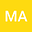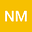$\mu$-Caputo type time-delay Langevin equations with two general fractional orders
••## Abstract

{In the present paper, a $\mu$-delayed Mittag-Leffler type function is introduced as a fundamental function. By means of $\mu$-delayed Mittag-Leffler type function, an exact analytical solution formula to non-homogeneous linear delayed Langevin equations involving two distinct $\mu$-Caputo type fractional derivatives of general orders is given. Also, a global solution of nonlinear version of delayed Langevin equations is inferred from the findings on hand and is verified with the aid of the functional(substitutional) operator. In terms of exponential function, we estimate $\mu$-delayed Mittag-Leffler type function. Existence uniqueness of solutions to nonlinear delayed Langevin fractional differential equations are obtained with regard to the weighted norm defined in accordance with exponential function. The notion of stability analysis in the sense of solutions to the described Langevin equations is discussed on the grounds of the fixed point approach. Numerical and simulated examples are shared to exemplify the theoretical findings. This paper provides novel results.
17 Mar 2022Submitted to Mathematical Methods in the Applied Sciences
18 Mar 2022Submission Checks Completed
18 Mar 2022Assigned to Editor
25 Mar 2022Reviewer(s) Assigned
30 Nov 2022Editorial Decision: Revise Minor